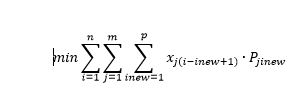# Decision Optimization

View Only

## CPLEX(default) cannot extract expression#### Archive UserSun January 21, 2018 06:48 AM#### ALEX FLEISCHERSun January 21, 2018 08:11 AM#### Archive UserMon January 22, 2018 01:52 AM#### ALEX FLEISCHERMon January 22, 2018 02:50 AM#### Archive UserMon January 22, 2018 09:51 PM#### ALEX FLEISCHERTue January 23, 2018 01:40 AM#### Archive UserTue January 23, 2018 03:52 AM#### Vaidik MiyaniMon March 21, 2022 03:43 PM#### Paul RubinMon March 21, 2022 05:36 PM#### Archive UserWed May 30, 2018 09:26 AM#### ALEX FLEISCHERWed May 30, 2018 02:42 PM#### Archive UserFri August 09, 2019 07:14 AM#### ALEX FLEISCHERMon September 02, 2019 02:06 AM#### Bastiaan DevileeWed April 28, 2021 11:33 AM#### Paul RubinMon May 03, 2021 04:45 PM#### Brandon Wymer PramanaThu July 15, 2021 09:15 AM#### ALEX FLEISCHERThu July 15, 2021 09:32 AM#### Brandon Wymer PramanaThu July 15, 2021 10:31 AM#### Mehdi El KrariThu February 17, 2022 03:54 PM#### Vincent BeraudierFri February 18, 2022 02:16 AM#### Mehdi El KrariFri February 18, 2022 10:40 AM#### ALEX FLEISCHERFri February 18, 2022 02:17 AM#### Mehdi El KrariFri February 18, 2022 10:42 AM• #### 1.  CPLEX(default) cannot extract expression

Posted Sun January 21, 2018 06:48 AM

Originally posted by: skyskyhuanghuang

Hi all,

I am a newbie in using OPL,i have written like this:

int M=...;
float P2=...;
float H1[1..M][1..M]=...;
float B0=...;
float n0=...;
dvar float+ Yji1[1..M][1..M]in 0..1 ;
//dvar int Yji1[1..M][1..M] in 0..1 ;

maximize
B0*sum(i in 1..M)(
log(1+sum(j in 1..M)(
P2*H1[j][i]*Yji1[j][i]/
(n0+sum(n,q in 1..M)P2*H1[n][i]*Yji1[n][q]
-P2*H1[j][i]*sum(k in 1..M)Yji1[j][k])
)
)/log(2)
);
When I run the code i get errors like:

CPLEX(default) cannot extract expression: maximize (sum(i in 1..3) (ln(sum(j in 1..3) ((H1[j][i]*0.126)*Yji1[j][i]) / (sum(n in 1..3, q in 1..3) (H1[n][i]*0.126)*Yji1[n][q]+((H1[j][i]*0.126)*(sum(k in 1..3) Yji1[j][k]))*(-1)+6.3e-13)+1) / 2.30258509299405) / (ln(2) / 2.30258509299405))*20000000. 20180120.mod /Solve 20:1-30:5 /home/sky/opl/Solve/20180120.mod OPL Problem Marker

When I remove the sum of noise power behind, the procedure is right, and this is why

maximize
B0*sum(i in 1..M)(
log(1+sum(j in 1..M)(
P2*H1[j][i]*Yji1[j][i]/n0
//(n0+sum(n,q in 1..M)P2*H1[n][i]*Yji1[n][q]
//-P2*H1[j][i]*sum(k in 1..M)Yji1[j][k])
)
)/log(2)
);

I dont know how to fix this or other problems in the code.

• #### 2.  Re: CPLEX(default) cannot extract expression

Posted Sun January 21, 2018 08:11 AM

Hi,

you get that error because log is not linear.

what you could do is use CPO.

See for example

using CP;

int scale=10000;

int M=2;
float P2=1.2;
float H1[i in 1..M][j in 1..M]=2;
float B0=1;
float n0=2;

//dvar int Yji1[1..M][1..M] in 0..1 ;

dvar int scaleYji1[1..M][1..M] in 0..scale ;
dexpr float Yji1[i in 1..M][j in 1..M]=scaleYji1[i][j]/scale;

maximize
B0*sum(i in 1..M)(
log(1+sum(j in 1..M)(
P2*H1[j][i]*Yji1[j][i]/
(n0+sum(n,q in 1..M)P2*H1[n][i]*Yji1[n][q]
-P2*H1[j][i]*sum(k in 1..M)Yji1[j][k])
)
)/log(2)
);

subject to
{

}

regards

• #### 3.  Re: CPLEX(default) cannot extract expression

Posted Mon January 22, 2018 01:52 AM

Originally posted by: skyskyhuanghuang

thank you very much.But after changing the calculation, time becomes longer, how to solve it?and the decision variable becomes scaleYji1,i just want decision variable to be Yji1.How can i sovle it ?

• #### 4.  Re: CPLEX(default) cannot extract expression

Posted Mon January 22, 2018 02:50 AM

Hi,

you may set a time limit

execute
{

cp.param.timelimit=60;
}

You may also stay with CPLEX but then you would have to linearize the log

regards

• #### 5.  Re: CPLEX(default) cannot extract expression

Posted Mon January 22, 2018 09:51 PM

Originally posted by: skyskyhuanghuang

Thanks for the code.

execute
{

cp.param.timelimit=60;
}

but for cplex,It does not seem to work.I don now why.Also, How to set the emphasismip parameter in the mod file to 1?

Thank you,

• #### 6.  Re: CPLEX(default) cannot extract expression

Posted Tue January 23, 2018 01:40 AM

Hi

with linear programming:

cplex.tilim=60;

cplex.mimpemphasis=1;

in an execute block

regards

• #### 7.  Re: CPLEX(default) cannot extract expression

Posted Tue January 23, 2018 03:52 AM

Originally posted by: skyskyhuanghuang

Thank you.

• #### 8.  RE: Re: CPLEX(default) cannot extract expression

Posted Mon March 21, 2022 03:43 PM
Hi,

int max_k=...;
int max_t=...;
range K=1..max_k;
range T=1..max_t;
int d[K][T]=...;
int p[K][T]=...;
int q[K][T]=...;
int h[K][T]=...;
int a[K]=...;
int M[K][T];
int C=...;
float e[K]=...;
dvar int x[K][T];
dvar int s[K][T];
dvar boolean y[K][T];
minimize sum(k in K,t in T)p[k][t]*x[k][t]+sum(k in K,t in T)q[k][t]*y[k][t]+sum(k in K,t in T)h[k][t]*s[k][t];
subject to {
forall(t in T, k in K){
s[k][t-1]+x[k][t]==d[k][t]+s[k][t];
}
forall(k in K){
s[k]==0;
}
forall(t in T, k in K){
x[k][t]<=M[k][t]*y[k][t];
}
forall(k in K, t in T){
M[k][t]==9999999999;
}
forall(t in T){
sum(k in K)e[k]*s[k][t]<=C;
}
}

------------------------------
Vaidik Miyani
------------------------------

• #### 9.  RE: Re: CPLEX(default) cannot extract expression

Posted Mon March 21, 2022 05:36 PM
In your first constraint, when t = 1 you refer to s[k], which does not exist.

------------------------------
Paul Rubin
Professor Emeritus
Michigan State University
------------------------------

• #### 10.  Re: CPLEX(default) cannot extract expression

Posted Wed May 30, 2018 09:26 AM

Originally posted by: MaxImal14582906

Hey,

I get the same error and i have a similar problem. My Optimization Problem looks like this:My code looks like this:

//parameters

int m=...; //number of machines

int n=...; //number of time steps in overall simulation

int p=...; // number of time steps in power cycle

float Pmin=...; //minimum of production

float Pmax=...; //maximum of production

range machines = 1..m;

range time = 1..n;

range ptime = 1..p;